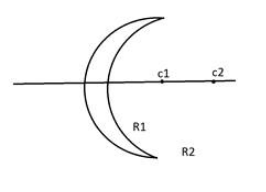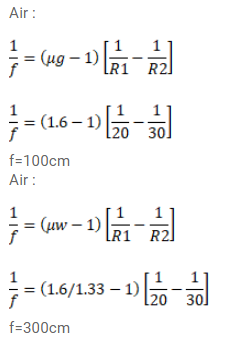# The radii of curvature of a lens areQuestion:

The radii of curvature of a lens are $20 \mathrm{~cm}$ and $30 \mathrm{~cm}$. The material of the lens has a refractive index $1.6$. Find the focal length of the lens (a) if it is placed in air, and (b) If it is placed in water (refractive index = 1.33).

$\mu \mathrm{w}$ lens refractive index $=\mu \mathrm{g}$Solution: